# Principal G-object

in a category

A concept in the theory of categories, instances of which are a principal fibre bundle in topology, a principal homogeneous space in algebraic geometry, etc. Letbe a group object in a categorywith products and final object. An objectis said to be a-object if there is given a morphism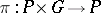for which the following diagrams are commutative: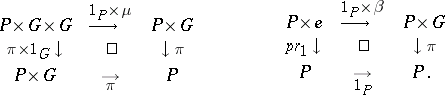Here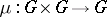is the group law morphism on, while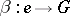is the unit element morphism into. More precisely, the-objects specified as above are called right-objects; the definition of left-objects is similar. As an example of a-object one may take the group objectitself, for whichcoincides with. This object is called the trivial-object. The-objects in the categoryform a category. The morphisms are morphism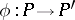ofwhich commute with(i.e. such that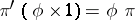). A-object is said to be a formal principal-object if the morphisms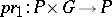andinduce an isomorphism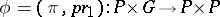. Ifis some Grothendieck topology on the category, a formal principal-objectis called a principal-object (with respect to the topology) if there exists a coveringof the final object such that for any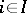the product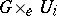is isomorphic to the trivial-object.

## Contents

### Examples.

1) Ifis the category of sets andis a group, then the non-empty-objects are called-sets. These are setsfor which a mapping() is defined such that for any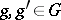one has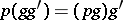, and for anyit is true that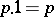. A principal-object is a-set in which for anythere exists a unique element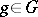such that(a principal homogeneous-set). Ifis not empty, the choice of a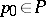determines a mapping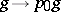which establishes an isomorphism betweenand the trivial-set. Thus, in any topology a formal principal-object is a principal-object.

2) Ifis a differentiable manifold andis a Lie group, then, takingto be the category of fibrations over, taking as group objectthe projection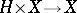, and defining a topology inwith the aid of families of open coverings, it is possible to obtain the definition of a principal-fibration.

Ifis a formal principal-object in a category, then for any objectin the category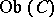the set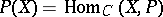is either empty or is a principal homogeneous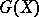-set.

A formal principal-objectis isomorphic to the trivial-object if and only if there exists a section. The set of isomorphism classes of formal principal-objects is denoted by. Ifis an Abelian group object, then the set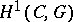, with the class of trivial-objects as a base point, is a group and can be computed by standard tools of homological algebra. In general, in the computation ofČech homology constructions are employed (cf. Non-Abelian cohomology).